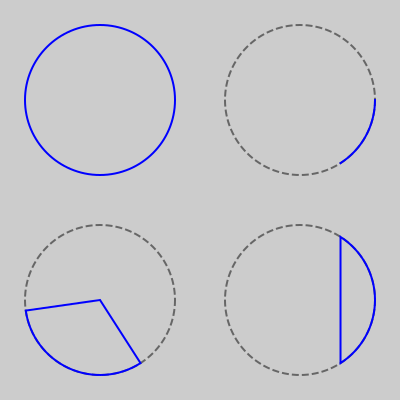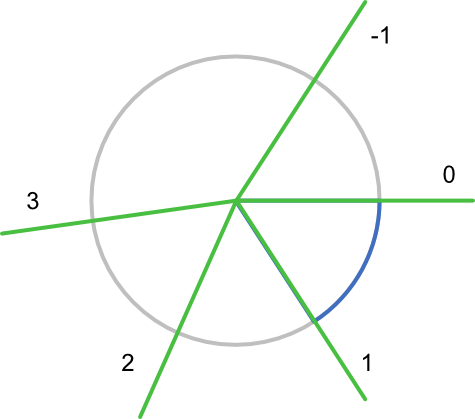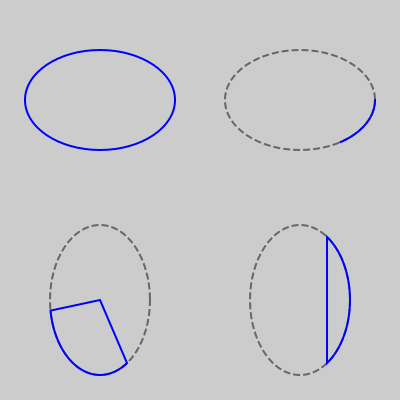# Circles and ellipses in generativepy

By Martin McBride, 2021-11-29
Tags: generativepy tutorial circle ellipse arc sector segment
Categories: generativepy generativepy tutorialThis tutorial shows how to create circular shapes in generativepy, using the geometry module. This includes the following shapes:

• Circles
• Ellipses
• Arcs
• Sectors
• Segments

For simplicity, the shapes are drawn as outlines. See the fill and stroke tutorial for details of how to fill and stroke shapes with different styles, and the patterns tutorial to learn about special fills such as gradients.

## Circle example code

Here is the code to create a circle, arc, sector, and segment :

from generativepy.drawing import make_image, setup
from generativepy.color import Color
from generativepy.geometry import Circle

def draw(ctx, pixel_width, pixel_height, frame_no, frame_count):

setup(ctx, pixel_width, pixel_height, background=Color(0.8))

blue = Color('blue')
grey = Color(0.4)
thickness = 2

make_image("circles-tutorial.png", draw, 400, 400)


This code is available on github in tutorial/shapes/circles.py.

Here is the resulting image:## Circle

This code, from the example above, draws a circle (top-left in the image above):

Circle(ctx).of_center_radius((100, 100), 75).stroke(blue, thickness)


The of_center_radius method creates a circle centred at (100, 100) with radius 75. These values are in user coordinates.

We stroke the circle with a blue line.

## Arc

This code draws an arc (top-right of the image above):

Circle(ctx).of_center_radius((300, 100), 75).stroke(grey, thickness, dash=)


The first line draws a grey, dashed circle. This is purely for illustration, it shows the full circle that the arc belongs to.

The second line draws the arc. An arc is part of the circumference of the circle. Drawing an arc is similar to drawing a circle, but we add a call to the as_arc method to create an arc. This method takes a start angle and an end angle. The arc is the sections of the circle between those angles (see the section Angles below).

We stroke the arc with a blue line. This is the circle in the top-left of the image above.

## Angles

The size of the arc is determined by the start and end angles, as illustrated by this diagram:Angles are measured clockwise from the horizontal (3 o'clock) position.

Angles are measured in radians. 1 radian is about 57.3 degrees (the exact figure is 180 divided by pi). So an angle of 1 radian from the start position is approximately 5 o'clock.

We can also use negative angles, which move anti-clockwise from the horizontal, so an angle of -1 radians is about 1 o'clock.

The arc example above has a start angle of 0 and an end angle of 1, so it produces the arc shown by the blue curve.

## Sector

This code draws a sector (bottom left of the image above):

Circle(ctx).of_center_radius((100, 300), 75).stroke(grey, thickness, dash=)


Again, the first line draws a grey, dashed circle as a reference.

The second line draws the sector, again outlined in blue. A sector is a pie slice shape. The shape is formed from an arc, but with the two ends of the arc joined to the centre of the circle. This time the angle range of 1 to 3 (see the angle diagram).

## Segment

This code draws a segment (bottom right of the image above):

  Circle(ctx).of_center_radius((300, 300), 75).stroke(grey, thickness, dash=)


This is similar to the sector. A segment is just an arc with the two ends of the arc joined by a straight line. The angle range is -1 to +1.

## Ellipse

Here is some example code to draw an ellipse, and also an elliptic arc, sector, and segment:

from generativepy.drawing import make_image, setup
from generativepy.color import Color
from generativepy.geometry import Ellipse

def draw(ctx, pixel_width, pixel_height, frame_no, frame_count):

setup(ctx, pixel_width, pixel_height, background=Color(0.8))

blue = Color('blue')
grey = Color(0.4)
thickness = 2

Ellipse(ctx).of_center_radius((300, 100), 75, 50).stroke(grey, thickness, dash=)
Ellipse(ctx).of_center_radius((300, 100), 75, 50).as_arc(0, 1).stroke(blue, thickness)

Ellipse(ctx).of_center_radius((100, 300), 50, 75).stroke(grey, thickness, dash=)
Ellipse(ctx).of_center_radius((100, 300), 50, 75).as_sector(1, 3).stroke(blue, thickness)

Ellipse(ctx).of_center_radius((300, 300), 50, 75).stroke(grey, thickness, dash=)
Ellipse(ctx).of_center_radius((300, 300), 50, 75).as_segment(-1, 1).stroke(blue, thickness)


This code is available on github in tutorial/shapes/ellipses.py.

Here is the resulting image:This code is very similar to the circles example, but we use the Ellipse object instead of a Circle object. The difference is that the of_center_radius has 3 parameters - the centre, x radius and the y radius. So:

of_center_radius((100, 100), 75, 50)


Gives an ellipse, centred at (100, 100), that is 150 wide and 100 high.